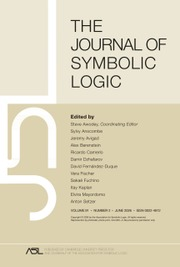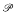HomeThe Journal of Symbolic Logic

# Dual weak pigeonhole principle, pseudo-surjective functions, and provability of circuit lower bounds

Published online by Cambridge University Press:  12 March 2014

Corresponding

## Abstract

This article is a continuation of our search for tautologies that are hard even for strong propositional proof systems like EF. cf. [14, 15]. The particular tautologies we study, the τ-formulas. are obtained from any/poly map g; they express that a string is outside of the range of g, Maps g considered here are particular pseudorandom generators. The ultimate goal is to deduce the hardness of the τ-formulas for at least EF from some general, plausible computational hardness hypothesis.

In this paper we introduce the notions of pseudo-surjective and iterable functions (related to free functions of ). These two properties imply the hardness of the τ-formulas from the function but unlike the hardness they are preserved under composition and iteration. We link the existence of maps with these two properties to the provability of circuit lower bounds, and we characterize maps g yielding hard τ-formulas in terms of a hitting set type property (all relative to a propositional proof system). We show that a proof system containing EF admits a pseudo-surjective function unless it simulates a proof system WF introduced by Jeřábek . an extension of EF.

We propose a concrete map g as a candidate function possibly pseudo-surjective or free for strong proof systems. The map is defined as a Nisan-Wigderson generator based on a random function and on a random sparse matrix. We prove that it is iterable in a particular way in resolution, yielding the output/input ratio n3 −ε (that improves upon a direct construction of Alekhnovich et al. ).

Type
Research Article
Information
The Journal of Symbolic Logic , March 2004 , pp. 265 - 286

## Access options

Get access to the full version of this content by using one of the access options below.

## References

Ajtai, M., The complexity of the pigeonhole principle, Proceedings of the IEEE 29th Annual Symposium on Foundation of Computer Science, 1988, pp. 346355.Google Scholar
Alekhnovich, M., Ben-Sasson, E., Razborov, A. A., and Wigderson, A., Pseudorandom generators in propositional proof complexity, Electronic Colloquium on Computational Complexity, vol. 23, 2000. abstract in Proceedings of the 41st Annual Symposium on Foundation of Computer Science, 2000, pp. 4353.Google Scholar
Atserias, A. and Bonet, M. L., On the automatizability of resolution and related propositional proof systems, 11th Annual Conference of the European Association for Computer Science Logic (CSL), Lecture Notes in Computer Science, vol. 2471, Springer-Verlag, 2002, pp. 569583.Google Scholar
Ben-Sasson, E. and Wigderson, A., Short proofs are narrow—resolution made simple, Proceedings of the 31st ACM Symposium on Theory of Computation, 1999, pp. 517526.Google Scholar
Buss, S. R., Impagliazzo, R., Krajíček, J., Pudlák, P., Razborov, A. A., and Sgall, J., Proof complexity in algebraic systems and bounded depth Frege systems with modular counting, Computational Complexity, vol. 6 (1996/1997), no. 3, pp. 256298.CrossRefGoogle Scholar
Cook, S. A., Feasibly constructive proofs and the propositional calculus, Proceedings of the 7th Annual ACM Symposium on Theory of Computing, ACM Press, 1975, pp. 8397.Google Scholar
Cook, S. A. and Reckhow, A. R., The relative efficiency of propositional proof systems, this Journal, vol. 44 (1979), no. 1, pp. 3650.Google Scholar
Goldreich, O., Candidate one-way functions based on expander graphs, Electronic Colloquium on Computational Complexity, vol. 90 (2000).Google Scholar
Goldreich, O., Goldwasser, S., and Micali, S., HOW to construct random functions, Journal of the ACM, vol. 33 (1986), no. 4, pp. 792807.CrossRefGoogle Scholar
Impagliazzo, R. and Wigderson, A., P = BPP unless E has sub-exponential circuits: derandomizing the XOR lemma, Proceedings of the 29th Annual ACM Symposium on Theory of Computing, 1997, pp. 220229.Google Scholar
Jeřábek, E., Dual weak pigeonhole principle, Boolean complexity, and derandomization, Annals of Pure and Applied Logic, to appear.Google Scholar
Krajíček, J., NO counter-example interpretation and interactive computation, Logic from Computer Science, Proceedings of a Workshop held November 13–17, 1989, in Berkeley (Moschovakis, Y. N., editor), Mathematical Sciences Research Institute Publication, vol. 21, Springer-Verlag, 1992, pp. 287293.Google Scholar
Krajíček, J., Bounded arithmetic, propositional logic, and complexity theory, Encyclopedia of Mathematics and its Applications, vol. 60, Cambridge University Press, 1995.CrossRefGoogle Scholar
Krajíček, J., On the weak pigeonhole principle, Fundamenta Mathematicae, vol. 170 (2001). no. 1-3, pp. 123140.CrossRefGoogle Scholar
Krajíček, J., Tautologies from pseudo-random generators, The Bulletin of Symbolic Logic, vol. 7 (2001), no. 2, pp. 197212.CrossRefGoogle Scholar
Krajíček, J., Hardness assumptions in the foundations of theoretical computer science, preprint in ITI series: http://iti.mff.cuni.cz/series/index.html, 01 2003.Google Scholar
Krajíček, J. and Pudlák, P., Propositional proof systems, the consistency of first order theories and the complexity of computations, this Journal, vol. 54 (1989), no. 3, pp. 10631079.Google Scholar
Krajíček, J. and Pudlák, P., Propositional provability in models of weak arithmetic, Computer Science Logic (Kaiserlautern, October '89) (Boerger, E., Kleine-Bunning, H., and Richter, M. M., editors). Lecture Notes in Computer Science, vol. 440, Springer-Verlag, 1990, pp. 193210.Google Scholar
Krajíček, J. and Pudlák, P., Some consequences of cryptographical conjectures forand EF, Information and Computation, vol. 140 (1998), no. 1, pp. 8294.CrossRefGoogle Scholar
Krajíček, J., Pudlák, P., and Sgall, J., Interactive computations of optimal solutions, Mathematical Foundations of Computer Science (B. Bystrica, August '90) (Rovan, B., editor). Lecture Notes in Computer Science, vol. 452, Springer-Verlag, 1990, pp. 4860.Google Scholar
Nisan, N. and Wigderson, A., Hardness versus randomness, Journal of Computer and System Sciences, vol. 49 (1994), pp. 149167.CrossRefGoogle Scholar
Paris, J. B. and Wilkie, A. J., Counting problems in bounded arithmetic, Methods in Mathematical Logic, Lecture Notes in Mathematics, vol. 1130, Springer-Verlag, 1985, pp. 317340.CrossRefGoogle Scholar
Paris, J. B., Wilkie, A. J., and Woods, A. R., Provability of the pigeonhole principle and the existence of infinitely many primes, this Journal, vol. 53 (1988), pp. 12351244.Google Scholar
Pudlák, P., Some relations between subsystems of arithmetic and the complexity of computations, Logic from Computer Science, Proceedings of a Workshop held November 13–17, 1989, in Berkeley (Moschovakis, Y. N., editor). Mathematical Sciences Research Institute Publication, vol. 21, Springer-Verlag, 1992, pp. 499519.Google Scholar
Pudlák, P., The lengths of proofs, Handbook of proof theory (Buss, S. R., editor), Elsevier, 1998. pp. 547637.CrossRefGoogle Scholar
Razborov, A. A., Pseudorandom generators hard for k-DNF resolution and polynomial calculus resolution, preprint, 05 2003.Google Scholar
Razborov, A. A., Unprovability of lower bounds on the circuit size in certain fragments of bounded arithmetic, Rossiĭskaya Akademiya Nauk, Seriya Matematicheskaya, vol. 59 (1995), no. 1, pp. 201224.Google Scholar
Razborov, A. A., Resolution lower bounds for perfect matching principles, Proceedings of the 17th IEEE Conference on Computational Complexity, 2002, pp. 2938.Google Scholar
Rudich, S., Super-bits, demi-bits, and ÑP/q poly-natural proofs, Proceedings of the 1st International Symposium on Randomization and Approximation Techniques in Computer Science, Lecture Notes in Computer Science, vol. 1269, Springer-Verlag, 1997, pp. 8593.CrossRefGoogle Scholar

### Full text views

Full text views reflects PDF downloads, PDFs sent to Google Drive, Dropbox and Kindle and HTML full text views.

Total number of HTML views: 0
Total number of PDF views: 11 *
View data table for this chart

* Views captured on Cambridge Core between September 2016 - 22nd January 2021. This data will be updated every 24 hours.

Hostname: page-component-76cb886bbf-r88h9 Total loading time: 0.441 Render date: 2021-01-22T11:00:40.758Z Query parameters: { "hasAccess": "0", "openAccess": "0", "isLogged": "0", "lang": "en" } Feature Flags: { "shouldUseShareProductTool": true, "shouldUseHypothesis": true, "isUnsiloEnabled": true, "metricsAbstractViews": false, "figures": false, "newCiteModal": false }

# Send article to Kindle

Note you can select to send to either the @free.kindle.com or @kindle.com variations. ‘@free.kindle.com’ emails are free but can only be sent to your device when it is connected to wi-fi. ‘@kindle.com’ emails can be delivered even when you are not connected to wi-fi, but note that service fees apply.

Find out more about the Kindle Personal Document Service.

Dual weak pigeonhole principle, pseudo-surjective functions, and provability of circuit lower bounds
Available formats
×

# Send article to Dropbox

To send this article to your Dropbox account, please select one or more formats and confirm that you agree to abide by our usage policies. If this is the first time you use this feature, you will be asked to authorise Cambridge Core to connect with your <service> account. Find out more about sending content to Dropbox.

Dual weak pigeonhole principle, pseudo-surjective functions, and provability of circuit lower bounds
Available formats
×

# Send article to Google Drive

To send this article to your Google Drive account, please select one or more formats and confirm that you agree to abide by our usage policies. If this is the first time you use this feature, you will be asked to authorise Cambridge Core to connect with your <service> account. Find out more about sending content to Google Drive.

Dual weak pigeonhole principle, pseudo-surjective functions, and provability of circuit lower bounds
Available formats
×
×